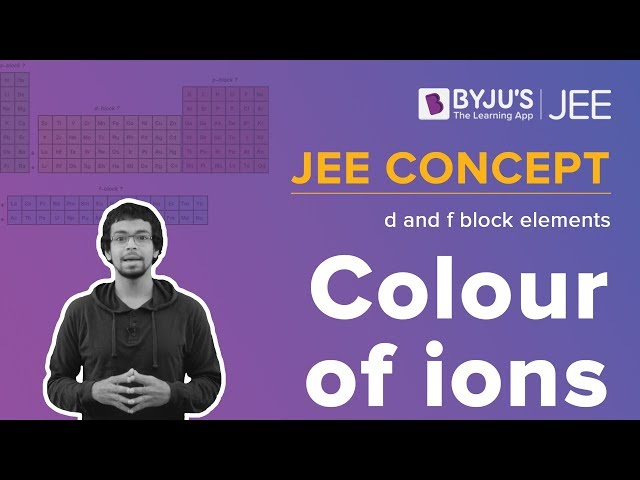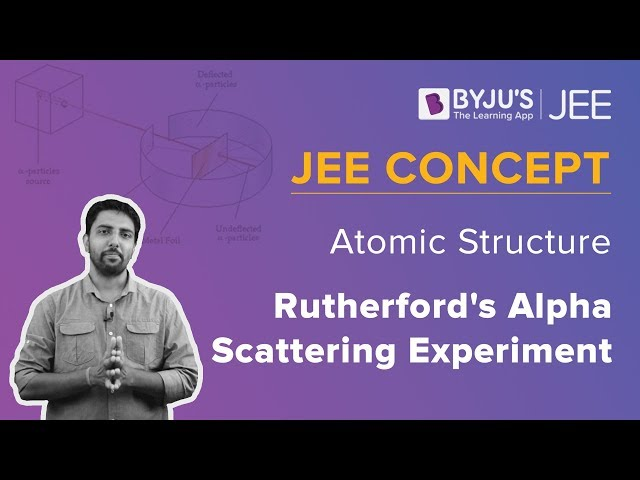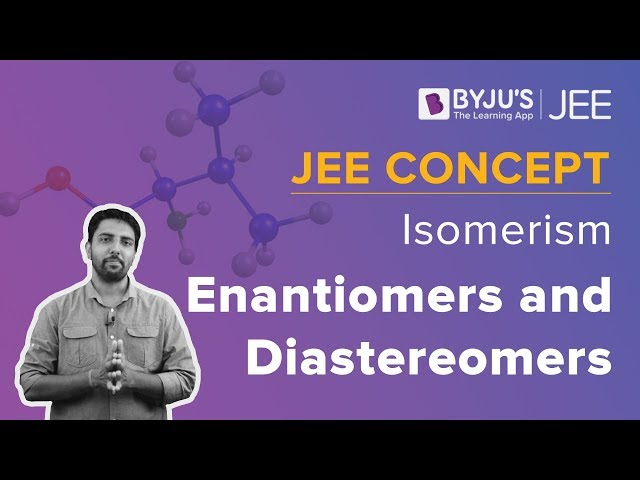Win up to 100% scholarship on Aakash BYJU'S JEE/NEET courses with ABNAT Win up to 100% scholarship on Aakash BYJU'S JEE/NEET courses with ABNAT

# JEE Advanced 2019 Chemistry Questions Paper 2

JEE Advanced Question Paper 2019 Chemistry Paper 2 is available here. Students can easily download these solutions from here in PDF format to practice offline. Practising these solutions will help students to improve their speed and accuracy, and they have an idea of what type of questions are asked from each chapter. Solving previous year question papers is an important aid for the students to analyze their performance. Students are advised to solve last year’s papers to crack the JEE Advanced.
Paper 2 – Chemistry
1. With reference to aqua regia, choose the correct option(s):
a) Aqua regia is prepared by mixing conc. HCl and conc. HNO3 in 3: 1 molar ratio.
b) Reaction of gold with aqua regia produces an anion having Au in +3 oxidation state.
c) Reaction of gold with aqua regia produces NO2 in the absence of air
d) The yellow colour of aqua regia is due to the presence of NOCl & Cl2.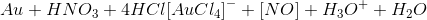a) Aqua regia is HCl & HNO3 (conc.) in a 3 : 1

b) Oxidation state of Au in [AuCl4]- is +3.

c) NOCl/NO is formed

d) NOCl is yellow in colour

2. Choose the correct option that gives aromatic compound as major product: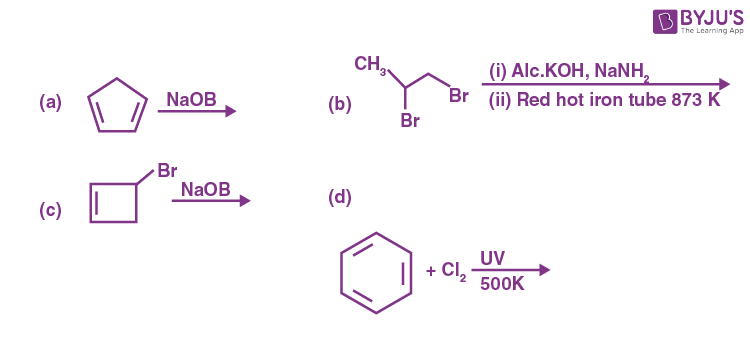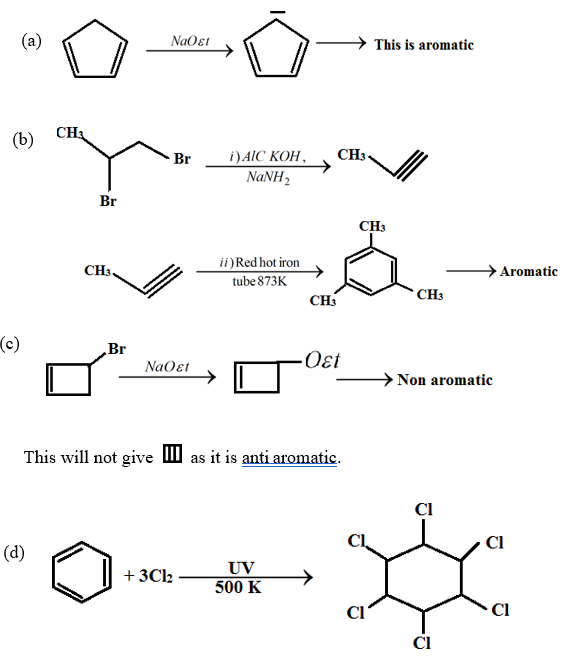3. Which of the following reaction produce propane as major product?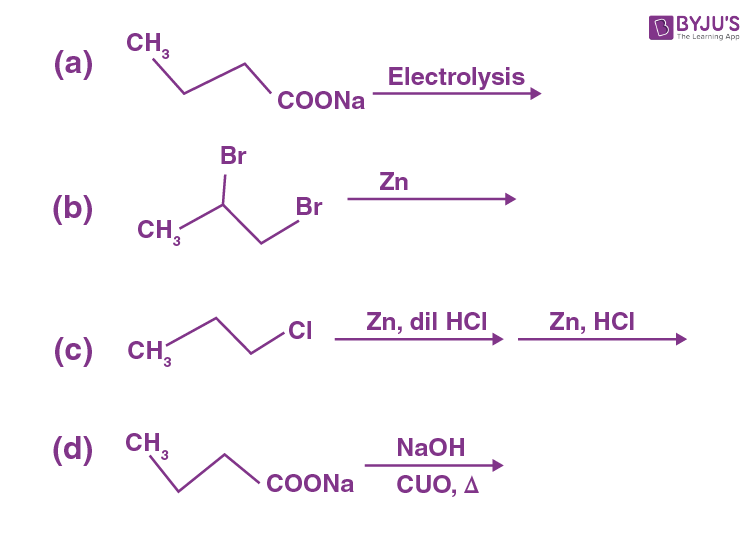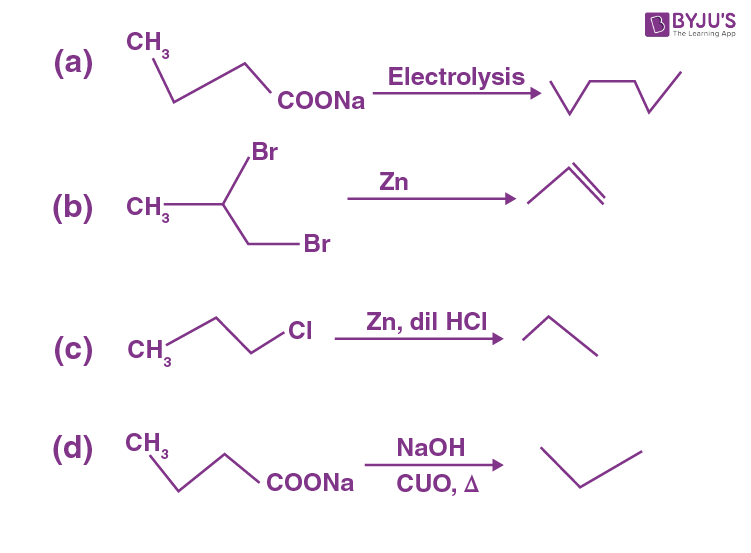4. Which of the following is/are correct
a) Teflon is formed by polymerization of tetrafluoroethene.
b) Natural rubber is the trans from of polyisoprene.
c) Cellulose contains only α-D-glucose linkage

a)Fact

b) Natural rubber is Cis form of polyisoprene

c) Cellulose contains B – 1, 4 – glycosidic linkage

d) Nylon 6 contains amide linkage.

Solution: (a, d)

5.Choose the correct option(s) for the following reaction sequence.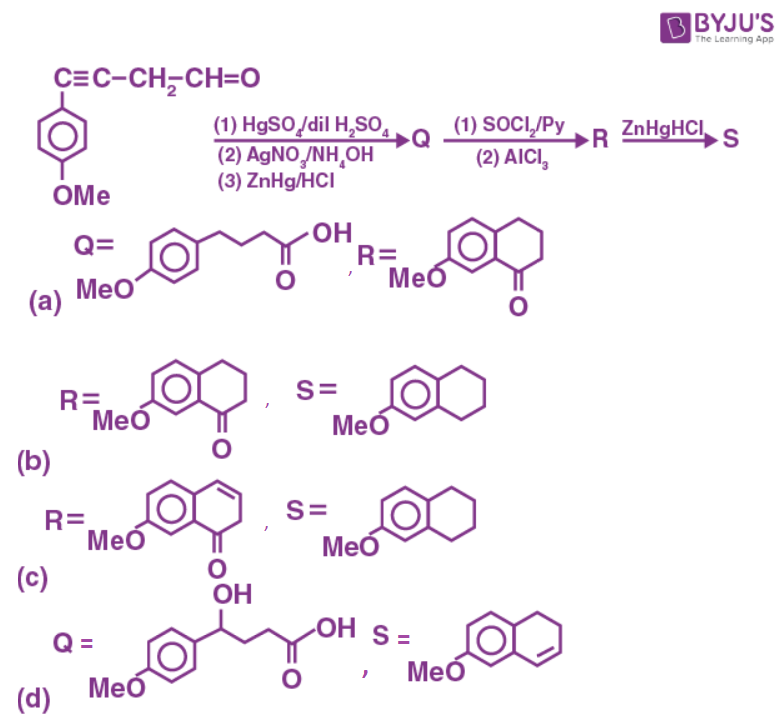Consider Q, R and S as major products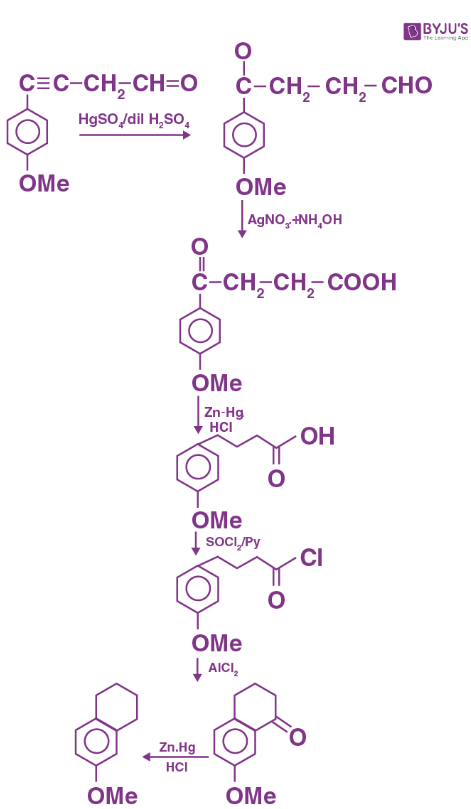6. Consider the following reaction(unbalanced)

Zn + Hot conc.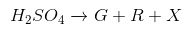Zn + conc.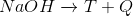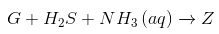(precipitate) X + Y

Choose the correct option(s)

a) R is a V-shaped molecule
b) Z is dirty white in colour
c) Bond order of Q is 1 in its ground state
d) The oxidation state of Zn in T is +1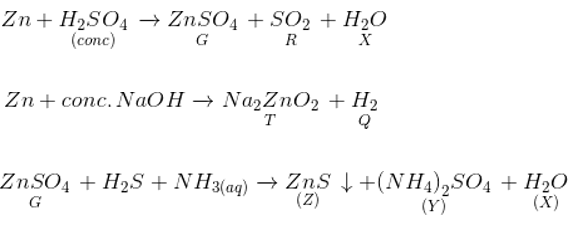a) SO2 is v shaped.

b) ZnS is dirty white in colour.

c) Bond order of H2 is 1.

d) Oxidation state of Zn in Na2ZnO2 is +2.

7. In the Mac. Arthur process of extraction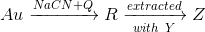a) R is [Au(CN4)](-)
b) Z is [Zn(CN)4]2-
c) Q is O2
d) Y is Zn

8. For He+ the electron is in orbit with energy equal to 3.4eV. The azimuthal quantum number for that orbit is 2 and magnetic quantum number is 0. Then which of the following is/are correct.
a) The subshell is 4d.
b) The number of angular nodes in it is 2.
c) The numbers of radial nodes in it is 3.
d) The nuclear charge experienced in n = 4 is 2e less than that in n = 1, where e is electric charge.

$$\begin{array}{l}E = E_0 \frac{z^2}{n^2}\end{array}$$

3.4 = 13.6 x 4/n2

n = 2

l = 2

a) Subshell is 4 d

b) Number of angular nodes is 2

c) Number of radial nodes is 1.

d) Nuclear charge would be the same.

9. Calculate the total number of cyclic ether (including stereo) having formula C4H8O.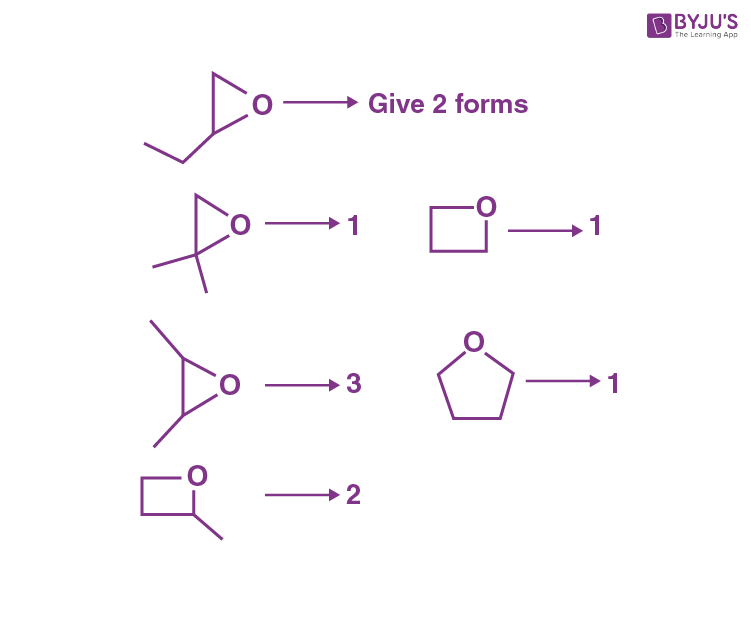Total 10

10. 1 mole of Rhombic sulphur is treated with conc. HNO3. Find the mass of H2O formed.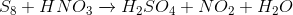Balancing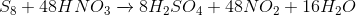∴ Mass of H2O = 288

11. Mole fraction of urea in 900 gram water is 0.05. Density of Solution is 1.2 g/cm3. Find molarity of Solution.

Number of moles of H2O = 900/18 = 50

n1/(n1+50) = 0.05

(n1 is No. of moles of urea)

=> n1 = 2.63

Weight of urea = 2.63 × 60 = 157.8 g

Total weight = 157.8 + 900 = 1057.8g

Volume = 1057.8/1.2 = 881.5 cm3

Molarity = 2.63/0.8 = 2.99

12. Number of hydroxyl group in compound ‘Y’ is: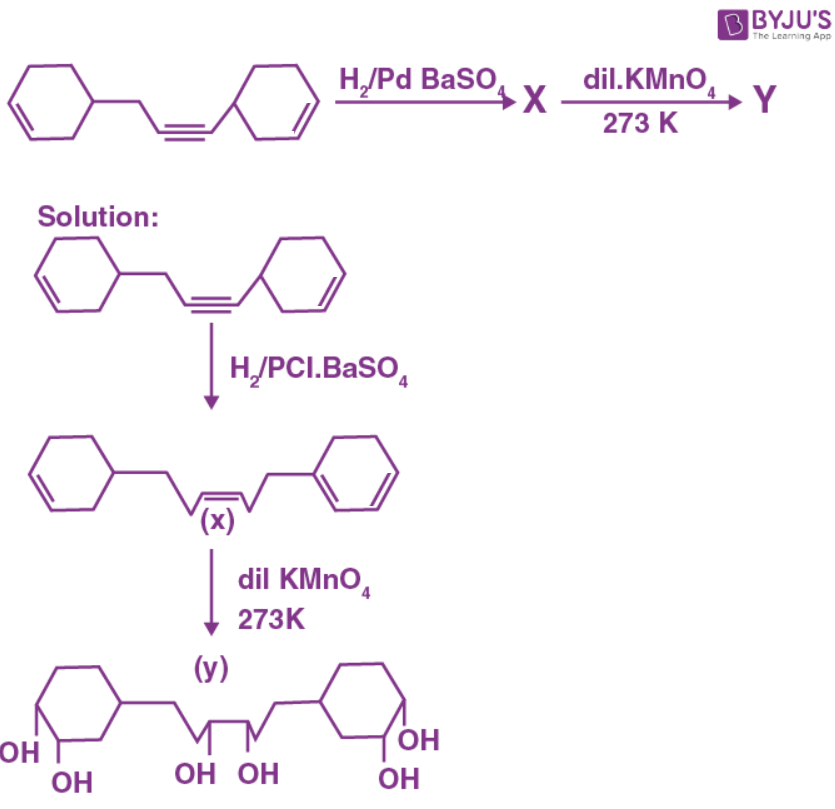Total 6 – OH groups.

13. In following reaction the value of K is 5 × 10-4 S-1.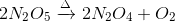Initial pressure was 1 atm, while the final pressure was 1.45 atm at time y × 103 sec calculate ‘y’.t = 0 1 0 0 t = t 1 – P P P/2 t = ∞ 0 1 0.5

P0 = 1 atm, Pt = 1.45 atm, P = 1 atm

$$\begin{array}{l}t = \frac{1}{2K}ln(\frac{P_{\infty} – P_0}{P_{\infty} – P_1})\end{array}$$

= 2.3 x 103

This implies, y = 2.3

14. Number of N-Mn-Cl bonds [N-Mn bonds is cis to Mn-Cl bond] in cis [Mn(en)2Cl2] are …….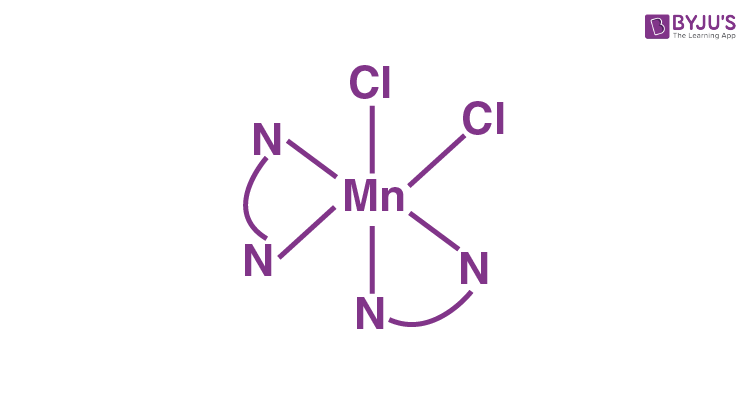This is the Cis form of [Mn(en)2Cl2]

Therefore, No. of N–Mn–Cl bonds = 6

15. Match the column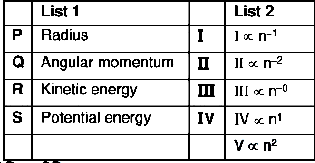Which of the following is correct

a) P I
b) P II
c) P V
d) P III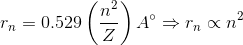16. Which of following is correct.a) S IV
b) R I
c) R II
d) S III

K.E. directly proportional to Z2/n2

Answer the question no. 17 & 18 on the basis of information given in Column – I & Column – II. Match the reactant in column – I with the possible intermediates and products of Column – II.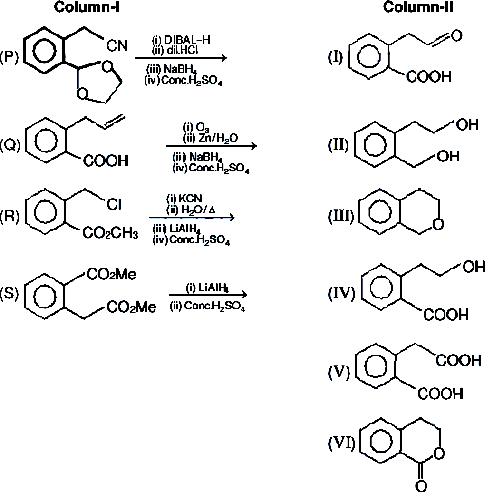17. Which of the following is correct?
a) P – II, III; S – II, III
b) P – II, IV; S – II, III
c) P – III, VI; S – II, III
d) P – I, III; S – IV, V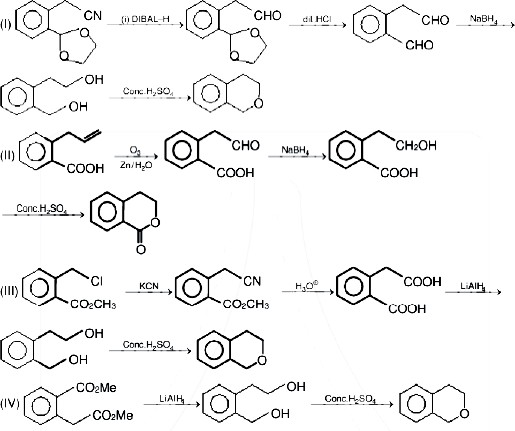18. Which of the following is correct?
a) Q – I, IV, VI; R – II, III, V
b) Q – I, III, VI; R – II, IV, V
c) Q – I, II, VI; R – II, III, VI
d) Q – I, IV, V; R – III, I, V